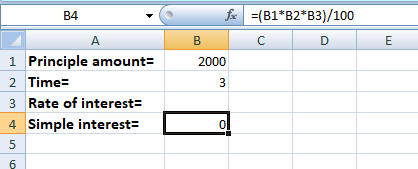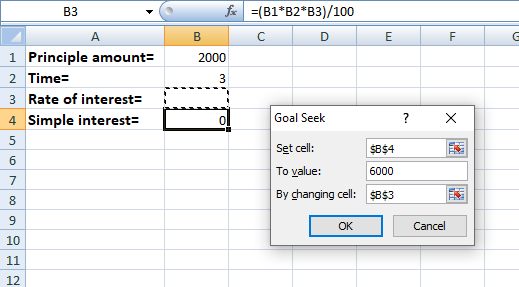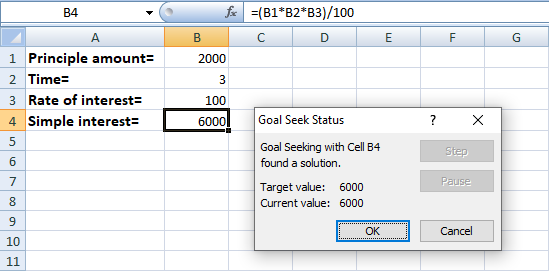# Goal Seek in Excel

• Last Updated : 15 May, 2021

Goal Seek is a tool available in MS EXCEL to determine or calculate any input value based on the formula and the output/resultant value.

To find the Goal seek :

Attention reader! Don’t stop learning now. If you are an Excel beginner (or an intermediate) and want to learn Excel, Geeksforgeeks brings the perfect course for you to start, Diving Into Excel

1. Go to Data Tab in MS EXCEL.
2. In the Data Tools group, you will find a What-if analysis.
3. Click on What if analysis, and you will find Goal seek.

Goal seek dialogue box has three parts:

1. Set cell: In this, we write the reference of the cell which contains the formula.
2. To value: In this, we write the value which we want to attain(or the given resultant value).
3. By changing cell: In this, we write the reference of the cell whose value we want to change(i.e. the cell whose value is to be calculated).

For example, we need to calculate the rate of interest when the time period, principal amount, and the simple interest are already known.

```Given : Principle amount = 2000
Time = 3 years
Simple interest = 6000```

### Using Goal Seek:

Follow the below steps to use Goal seek functionality in Excel:

• Open the MS EXCEL and insert the values.
• Write the formula of simple interest in any one cell (B4).• Open the Goal seek dialogue box.
• In the Set Cell box select the cell which contains the formula of simple interest (B4).
• In the To Value box write the resultant value of the simple interest i.e. 6000 (Given in question).
• In the By Changing Cell write the reference of the cell in which you want the rate of interest value (B3).• Click OK.
• The Rate of interest value is calculated in the cell (B3).My Personal Notes arrow_drop_up